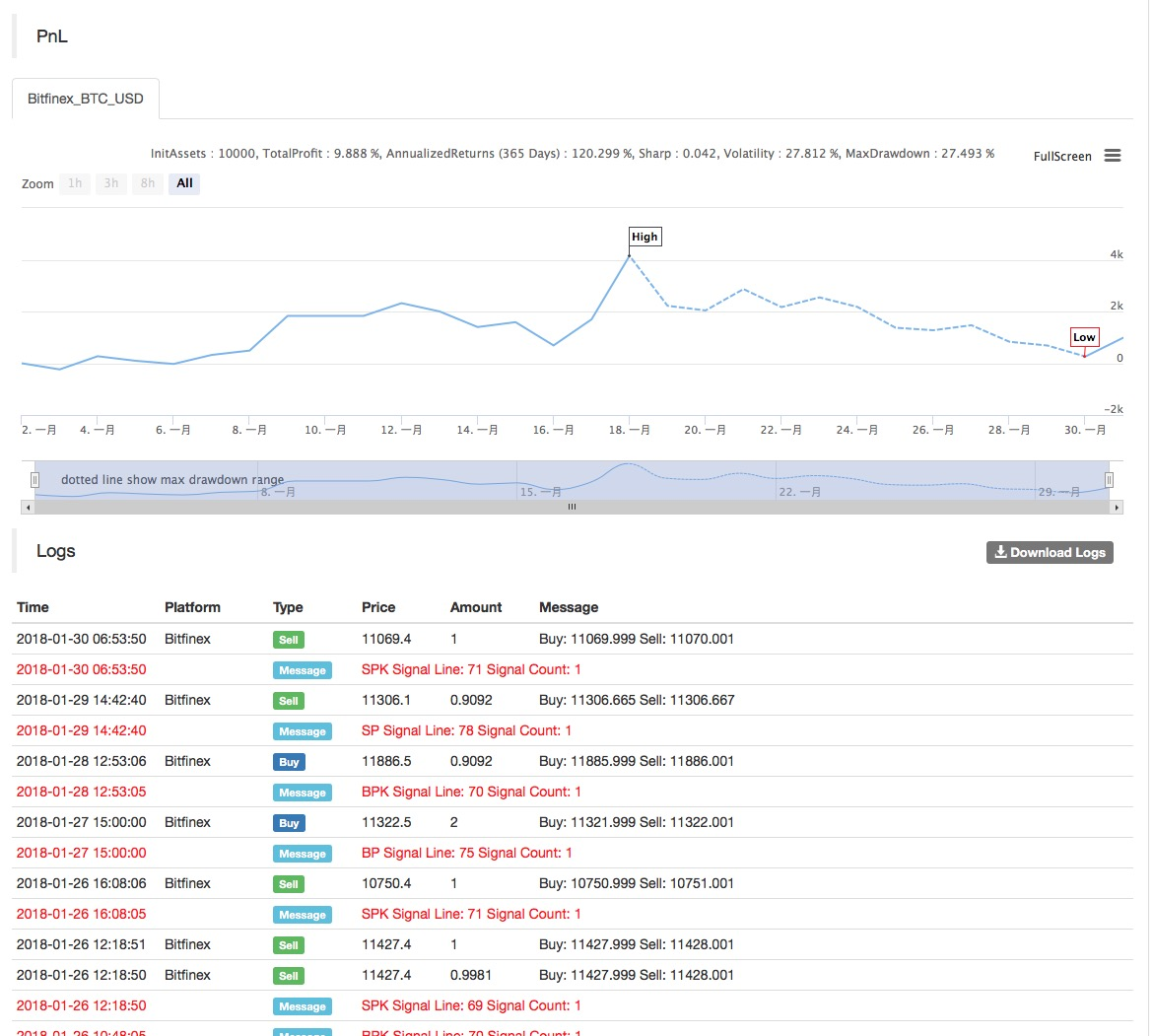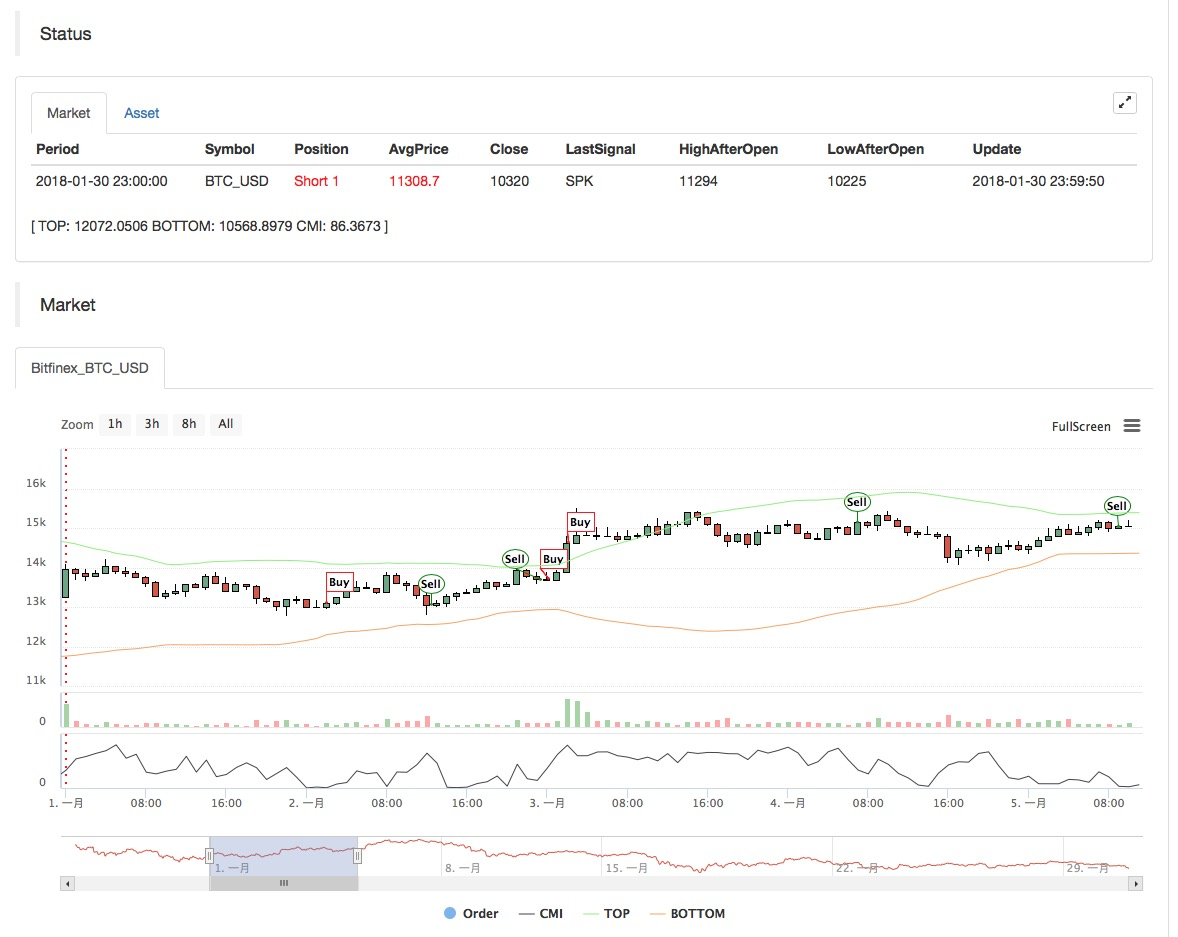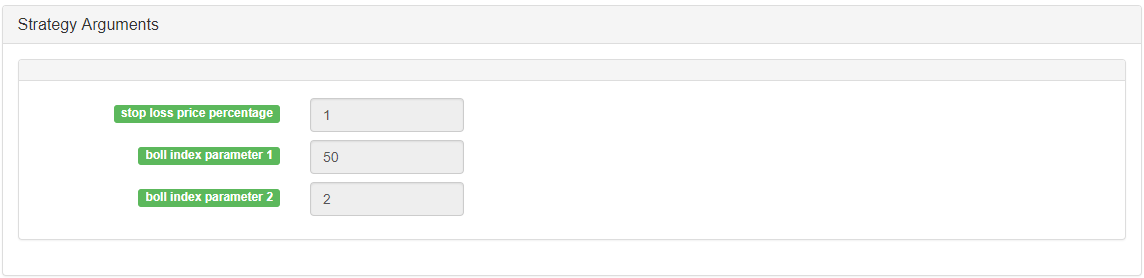Thermostat Strategy using on crypto market by MyLanguage

Author: , Created: 2020-08-21 19:19:20, Updated: 2020-08-21 19:20:01

Data cycle: 1H

Support: Commodity Futures, Digital Currency Futures, Digital Currency Spot• Main chart: upper track, formula: TOP^^MAC+N_TMPTMP; / / upper track of boll lower track, formula: BOTTOM^^MAC-N_TMPTMP;//lower track of boll

• Secondary chart: CMI, formula: CMI: ABS(C-REF(C,N_CMI-1))/(HHV(H,N_CMI)-LLV(L,N_CMI))*100; //0-100 the larger the value, the stronger the trend, CMI <20 is oscillation, CMI>20 is trendSource code:

(*backtest
start: 2018-11-06 00:00:00
end: 2018-12-04 00:00:00
period: 1h
exchanges: [{"eid":"Futures_CTP","currency":"FUTURES"}]
*)

MAC:=MA(CLOSE,N);
TMP:=STD(CLOSE,N);
TOP^^MAC+N_TMP*TMP;// upper track of boll
BOTTOM^^MAC-N_TMP*TMP;// lower track of boll
BBOLL:=C>MAC;
SBOLL:=C<MAC;
N_CMI:=30;

CMI:ABS(C-REF(C,N_CMI-1))/(HHV(H,N_CMI)-LLV(L,N_CMI))*100;
//0-100 the larger the value, the stronger the trend, CMI <20 is oscillation mode, CMI>20 is the trend

N_KD:=9;
M1:=3;
M2:=3;
RSV:=(CLOSE-LLV(LOW,N_KD))/(HHV(HIGH,N_KD)-LLV(LOW,N_KD))*100;
//(1)closing price - the lowest of cycle N, (2)the highest of cycle N - the lowest of cycle N, (1)/(2)
K:=SMA(RSV,M1,1);//MA of RSV
D:=SMA(K,M2,1);//MA of K
MIND:=30;
BKD:=K>D AND D<MIND;
SKD:=K<D AND D>100-MIND;

//oscillation mode
SELLPK1:=CMI < 20 AND SKD;//if it's oscillation, sell to close long position and sell short to open position immediately

//Disposal of the original oscillating position under the trend mode
SELLY1:=REF(CMI,BARSBK) < 20 AND C>BKPRICE*(1+0.01*STOPLOSS*3) AND K<D;//if it's oscillation, long position take profit
BUYY1:=REF(CMI,BARSSK) < 20 AND C<SKPRICE*(1-0.01*STOPLOSS*3) AND K>D;//if it's oscillation, short position take profit

//trend mode
SELLPK2:=CMI >= 20 AND C < BOTTOM;//if it's trend, sell to close long position and sell short to open position immediately

//Disposal of the original oscillating position under the trend mode
SELLY2:=REF(CMI,BARSBK) >= 20 AND C>BKPRICE*(1+0.01*STOPLOSS*3) AND SBOLL;//if it's trend, long position take profit
BUYY2:=REF(CMI,BARSSK) >= 20 AND C<SKPRICE*(1-0.01*STOPLOSS*3) AND BBOLL;//if it's trend, short position take profit

SELLS2:=REF(CMI,BARSBK) >= 20 AND C<BKPRICE*(1-0.01*STOPLOSS) AND SBOLL;//if it's trend, long position stop loss
BUYS2:=REF(CMI,BARSSK) >= 20 AND C>SKPRICE*(1+0.01*STOPLOSS) AND BBOLL;//if it's trend, short position stop loss

IF BARPOS>N THEN BEGIN
SELLPK1,SPK;